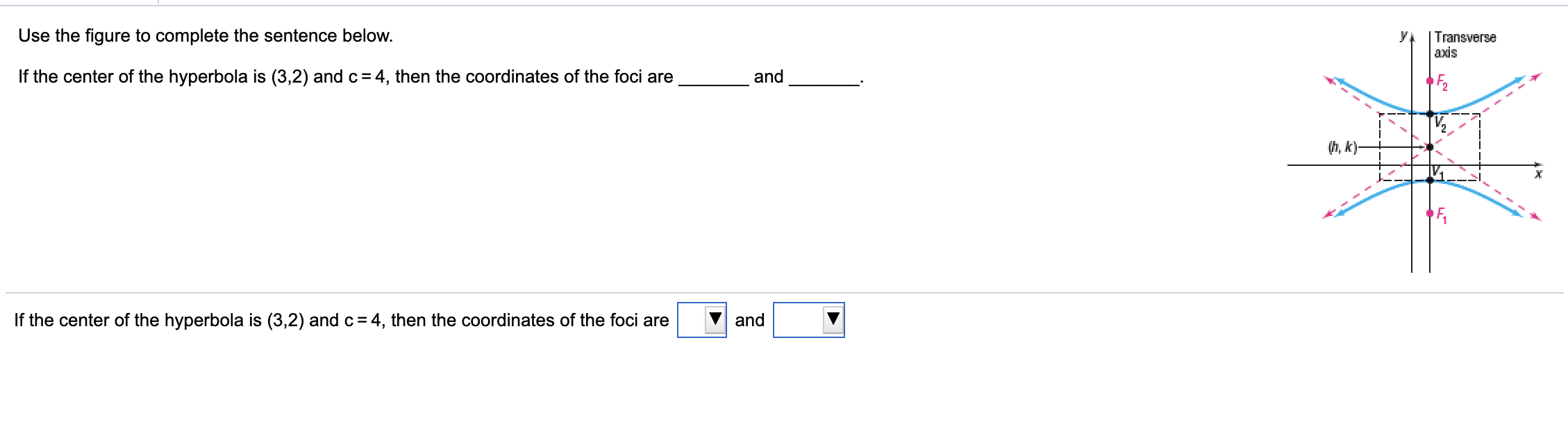# Use the figure to complete the sentence below. y. | Transverse axis If the center of the hyperbola is (3,2) and c=4, then the coordinates of the foci are and (h, k)- If the center of the hyperbola is (3,2) andc=4, then the coordinates of the foci are and

Questionhelp_outlineImage TranscriptioncloseUse the figure to complete the sentence below. y. | Transverse axis If the center of the hyperbola is (3,2) and c=4, then the coordinates of the foci are and (h, k)- If the center of the hyperbola is (3,2) andc=4, then the coordinates of the foci are and fullscreen